# Neutron Scattering VisualizationDate: 11/14/2019

As of the date shown above, I work for Dr. Kate Ross on a condensed matter physics team at Colorado State University. As part of my work, I was asked to create a data visualization tool for a Neutron Scattering Experiment conducted by the graduate student Gavin Hester. The plan is to build this tool using Python and the Dash Framwork. The Dash Framwork integrates Python's Plotly API with the Flask web hosting library.

• I was asked to: create a heat map that we can be scanned over, such that, viewers can interactively look at different cross sections of the heat map.

### What Is This Notebook For?¶

• In order to start building this application, I need to first prototype some plots in Plotly. Than, I can build the Dash visualization application that makes use of these plots.
• There is an additional problem. I was given relatively little information as to how the data will be presented, and so, before I get started on prototyping plots, I will first investigate the data file that I will be using. I was told that the file contains points that form a grid with associated intensity values from a Neutron Scattering experiment, but I was not informed how exactly this information is presented in the file.

### Note:¶

In this notebook I have created some plotly plots. As a result, In order to run this notebook, you may need to install the plotly-extension for jupyterlab or jupyter notebook (depending on which program you are using).

## Configuring The Notebook¶

### Imports¶

In :
# General Processing
import pandas as pd
import numpy as np

# Visualization
import plotly.graph_objs as go
import plotly.express as px
import plotly.io as pio
import matplotlib.pyplot as plt

# Standard Library
from typing import List


### Setting Display Options¶

In :
%matplotlib inline
np.set_printoptions(precision=2, linewidth=150)


The code below allows for the user to create a display object for displaying multiple pandas dataframes next to one another.

In :
class display(object):
"""Display HTML representation of multiple objects"""
template = """<div style="float: left; padding: 10px;">
<p style='font-family:"Courier New", Courier, monospace'>{0}</p>{1}
</div>"""
def __init__(self, *args: List[pd.DataFrame]):
self.args = args

def _repr_html_(self):
return '\n'.join(self.template.format(a, pd.DataFrame(eval(a))._repr_html_())
for a in self.args)

def __repr__(self):
return '\n\n'.join(a + '\n' + repr(eval(a))
for a in self.args)


## Inspect The Data¶

Define the data file to analyze

In :
data_file = "../data/1K0Slice_Integratedpm0p1.csv"


In :
df = pd.read_csv(data_file,names=["x","y","z"])


Size of the Data

In :
rows, columns = df.shape
print("Data Rows:   ", rows)
print("Data Columns:", columns)

Data Rows:    36461
Data Columns: 3


Inspect Elements

In :
first_five_elements = df.head()
last_five_elements = df.tail()
display("first_five_elements", "last_five_elements")

Out:

first_five_elements

x y z
0 -1.7929 0.002324 10.0610
1 -1.7851 0.002324 10.3220
2 -1.7748 0.002324 9.8859
3 -1.7652 0.002324 9.6727
4 -1.7552 0.002324 9.5339

last_five_elements

x y z
36456 1.7640 0.99742 0.0
36457 1.7738 0.99742 0.0
36458 1.7837 0.99742 0.0
36459 1.7932 0.99742 0.0
36460 1.8024 0.99742 0.0

Get the unique element counts

In :
for key in df:
print(f"unique {key} count:", df[key].unique().size)

unique x count: 361
unique y count: 101
unique z count: 26894

• From the information I was given, and based on how few unique values there are in x and y, I am guessing that the x and y values form a grid of points and the z values represent the intensities in the Neutron Scattering Data.

Let's Check!

### Plotting Raw Data¶

I am going to make some plots of the raw data to see if I can make sense of it.

#### x-data¶

In :
plt.scatter(range(len(df.x)), df.x, s=1, marker='o');
plt.title("X-Data")
plt.ylabel("x-value")
plt.xlabel("x-index")
plt.show()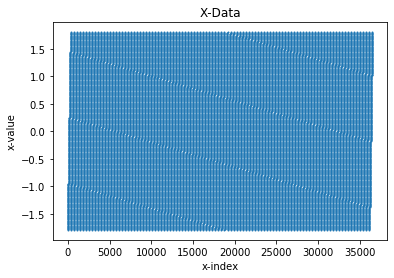• There is clearly a grid of coordinates, lets plot a smaller range to see if we can make sense of this.
In :
plt.scatter(range(2000),df.x[:2000],s=1,marker='o');
plt.xlabel("x-index")
plt.ylabel("x-value")
plt.title("X-Data (First 2k Values)")
plt.show()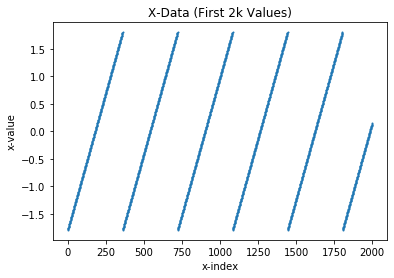• This plot is much more illuminating. As can be seen in the plot, there is a pattern of linearly spaced x coordinates that get repeated roughly every 300 elements. This interval likely occures every 361 elements because that's how many unique x elements I found earlier!

LETS CHECK!

If this is true, than every grouping of every 361 elements will be the same as the first grouping of 361 elements.

In :
same_elements = True
for i,val in enumerate(df.x):
if val != df.x[i % 361]:
same_elements = False
print(same_elements)

True


Now I will inspect the range of the x coordinates.

In :
print(f"Range(x) = [{df.x.unique().min()},{df.x.unique().max()}]")

Range(x) = [-1.7929,1.8024]


#### y-data¶

In :
plt.scatter(range(len(df.y)), df.y, s=1, marker='o');
plt.xlabel("y-index")
plt.ylabel("y-value")
plt.title("Y-Data")
plt.show()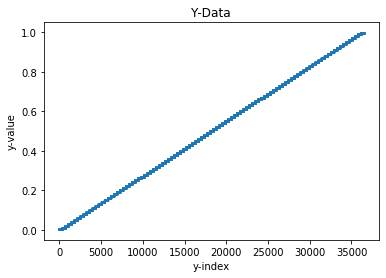• If your look closely, you can see that the y values seem to have a stepwise pattern, but lets make a smaller plot to verify this.
In :
plt.scatter(range(2000), df.y[:2000], s=1, marker='o');
plt.xlabel("y-index")
plt.ylabel("y-value")
plt.title("Y-Data (First 2k Values)")
plt.show()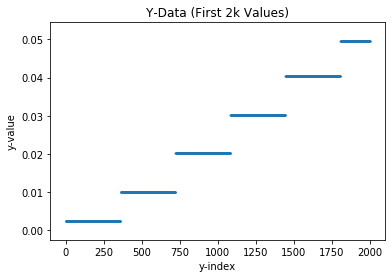• Sure Enough, y seems to stay constant for roughly every 300 values. It appears that in our data, a particular y value is chosen and than roughly 300 unique x elements are chosen.

Let's Check!

In :
all_counts = [] # consecutive counts associated with each unique element
curr = df.y  # current unique element
curr_count = 0  # count of the number of consecutive times I have seen curr
for val in df.y:
if curr == val:
curr_count += 1
else:
curr = val
all_counts.append(curr_count)

curr_count = 1

# Check that the number of uniqu
print("Did all unique consecutive elements show up the same number of times?", len(set(all_counts)) == 1)
print("How many times did each unique element show up consecutively?", all_counts.pop())

Did all unique consecutive elements show up the same number of times? True
How many times did each unique element show up consecutively? 361


Now I will inspect the range of the y coordinates.

In :
print(f"Range(x) = [{df.y.unique().min()},{df.y.unique().max()}]")

Range(x) = [0.0023244,0.9974200000000001]


#### x vs. y data¶

In :
%matplotlib inline
plt.scatter(df.y,
df.x,
s=5,
c=np.arange(len(df.x)) // len(df.x.unique()),
marker='o');
plt.colorbar()
plt.xlabel("y-data")
plt.ylabel("x-data")
plt.title("X-Data Vs. Y-Data");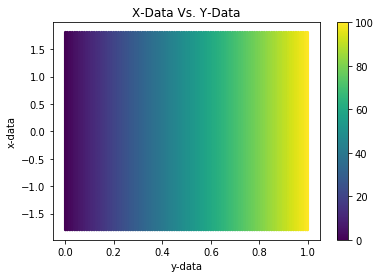• The scatter plot above starts using a different color every 361 elements. The plot above is further indication that the x and y values form a grid for the z value intensity data.

#### z-data¶

In :
plt.scatter(range(len(df.z)), df.z,s=1)
plt.xlabel("Z index")
plt.ylabel("z-data")
plt.title("z-Data");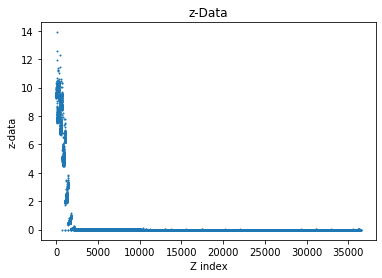• This data seems rather odd at a first glance. I asked Danielle Harris about what was going on here (she is another graduate student that also works on Neutron Scattering Experiments) and she explained to me that this was normal behavior. She explained that those strange values we are observing come from the elastic line, that is washing out the rest of the data.

#### Conclusion¶

Now that I feel comfortable saying that the x and y values are grid coordinates for the intensity values that are represented by z, let's go ahead and make some plots!

# Plots¶

### Scatter¶

To get a better grasp on the data, I will go ahead and make a scatter plot.

In :
data = [go.Scatter3d(x=df.x, y=df.y, z=df.z,
mode="markers",
marker = dict(